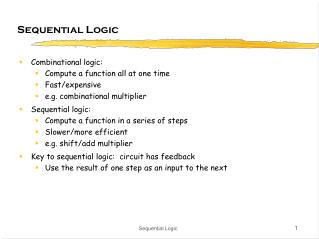Download PresentationSequential Logic

# Sequential Logic - PowerPoint PPT Presentation

Sequential Logic. Combinational logic: Compute a function all at one time Fast/expensive e.g. combinational multiplier Sequential logic: Compute a function in a series of steps Slower/more efficient e.g. shift/add multiplier Key to sequential logic: circuit has feedbackI am the owner, or an agent authorized to act on behalf of the owner, of the copyrighted work described.
Download Presentation## Sequential Logic

Download Policy: Content on the Website is provided to you AS IS for your information and personal use and may not be sold / licensed / shared on other websites without getting consent from its author.While downloading, if for some reason you are not able to download a presentation, the publisher may have deleted the file from their server.

- - - - - - - - - - - - - - - - - - - - - - - - - - E N D - - - - - - - - - - - - - - - - - - - - - - - - - -
Presentation Transcript
1. Sequential Logic • Combinational logic: • Compute a function all at one time • Fast/expensive • e.g. combinational multiplier • Sequential logic: • Compute a function in a series of steps • Slower/more efficient • e.g. shift/add multiplier • Key to sequential logic: circuit has feedback • Use the result of one step as an input to the next Sequential Logic

2. Circuits with feedback • How to control feedback? • what stops values from cycling around endlessly? • this is an asynchronous sequential circuit X1X2•••Xn Z1Z2•••Zn Combinational circuit Sequential Logic

3. "1" "stored value" "0" "remember" "load" "stored value" "data" Simplest circuits with feedback: latch • Two inverters form a static memory cell • will hold value as long as it has power applied • How to get a new value into the memory cell? • selectively break feedback path • load new value into cell Sequential Logic

4. Let’s Use This Latch • What happens? X1X2•••Xn Z1Z2••• Combinational circuit Sequential Logic

5. What We Need: • When inputs change... • Wait until combinational logic has finished and result it stable... • Then sample the output value and save... • Feed the saved output back to the input of the combinational logic • Make sure the saved output can’t change • Key idea: we sample the result at the right time, i.e. when it is ready • Only then do we update the stored value • How do we know when to sample? • How do we know when the inputs changed? • How do we know how long to wait? Sequential Logic

6. D Q What We Need: • A circuit that can sample a value • A signal that says when to sample • Edge-triggered D flip-flop (register) • Samples on positive edge of clock • Holds value until next positive edge • Most common storage element • Clock • Periodic signal, each rising edge signals D flip-flops to sample • All registers sample at the same time Sequential Logic

7. D Q Let’s Use This D flip-flop • Does this work? • What do we need to say about the inputs X1, X2, ...? • This is a synchronous sequential circuit X1X2•••Xn Z1Z2••• Combinational circuit Sequential Logic

8. D D Q Q Registers • Sample data using clock • Hold data between clock cycles • Computation (and delay) occurs between registers data in data out clock stable may change data in clock stable stable stable data out (Q) Sequential Logic

9. Example - Circuit with Feedback • Output is a function of arbitrarily many past inputs Sequential Logic

10. B A outt outt+1– 0 0 0– 0 1 10 1 – 01 1 – 1 Examples (cont’d) • Output is a function of inputs and the previous state of the circuit Sequential Logic

11. Example - Circuit without Feedback • Output is a function of the input sampled at three different points in time Sequential Logic

12. D D Q Q Tsu Th input clock Timing Methodologies (cont’d) • Definition of terms • setup time: minimum time before the clocking event by which the input must be stable (Tsu) • hold time: minimum time after the clocking event until which the input must remain stable (Th) data clock there is a timing "window" around the clocking event during which the input must remain stable and unchanged in order to be recognized stable changing data clock Sequential Logic

13. Tsu2ns Th1ns D Tsu2ns Th1ns CLK Tw 7ns Q Tplh [2,4]ns Tphl[1,3]ns Typical timing specifications • Positive edge-triggered D flip-flop • setup and hold times • minimum clock width • propagation delays (low to high, high to low, max and typical) all measurements are made from the clocking event that is, the rising edge of the clock Sequential Logic

14. Synchronous System Model • Register-to-register operation • Perform operations during transfer • Many transfers/operations occur simultaneously Sequential Logic

15. System Clock Frequency • Register transfer must fit into one clock cycle • reg tpd + C.L. tpd + reg tsu < Tclk • Use maximum delays • Find the “critical path” • Longest register-register delay Sequential Logic